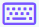:

Easy
Medium
Hard

## Integral calculator with steps

Antiderivative (Integral) calculator is an online tool used to calculate the antiderivatives with steps. This integral calculator integrates the functions w.r.t a variable i.e., x, y, z, u, or t.

This integration calculator evaluates the expressions of the indefinite integral as well as the definite integral with steps.

In the case of definite integral, this integral calculator uses the upper and lower limits of the given function. The upper and lower limits are the maximum and minimum values of intervals.

## calculator work?

You can evaluate the integrals by following the steps below.

• Choose the method i.e., definite or indefinite.
• Select the variable.
• Fill the upper and lower limits boxes in case of the definite integral.
• Write the function into the input box.
• Use the keypad iconto write math keys.
• Click the calculate button. You will get the output of the given function with a step-by-step calculation.
• Hit the reset button to enter a new function.

## What is an integral?

In mathematics, an integral assigns numbers to functions in a way that describes the area, volume, displacement, and other concepts that arise by combining infinitesimal data. The process of finding the integral is known as integration.

In the equation of integral three terms are used:

1. Integration symbol
2. Integrant (function to be integrated)
3. Variable of integration

The equation of integral is given below.

$$\int f\left(x\right)dx$$

Types of integrals are:

### Rules of integration

Some rules of integration are listed in the table.

 Names Rules Constant rule $$\int \left(a\right)dx=ax+c$$ Power rule $$\int x^ndx=\frac{x^{n+1}}{n+1}+c$$ Difference rule $$\int \left(u-v\right)dx=\int \left(u\right)dx-\int \left(v\right)dx$$ Sum rule $$\int \left(u+v\right)dx=\int \left(u\right)dx+\int \left(v\right)dx$$ Multiplication by a constant $$\int af\left(x\right)dx=a\int f\left(x\right)dx$$ Variable rule $$\int xdx=\frac{x^2}{2}+c$$

## How to evaluate the integrals?

By using definite and indefinite integrals we can easily evaluate the antiderivatives of the given functions. Below are some examples that are evaluated by our integral calculator.

Example 1: For indefinite integral

Evaluate $$\int \left(9x+3\right)dx$$

Solution

Step 1: Apply the sum and multiplication by a constant rule.

$$\int \left(9x+3\right)dx=\int \left(9x\right)dx+\int \left(3\right)dx$$

$$\int \left(9x+3\right)dx=9\int \left(x\right)dx+3\int dx$$

Step 2: Now apply the power and constant rule.

$$\int \left(9x+3\right)dx=9\left(\frac{x^{1+1}}{1+1}\right)+3\left(x\right)+c$$

$$\int \left(9x+3\right)dx=9\left(\frac{x^2}{2}\right)+3x+c$$

$$\int \left(9x+3\right)dx=\frac{9x^2}{2}+3x+c$$

Example 2: For definite integral

Evaluate $$\int _1^3\left(2x\right)dx$$

Solution

Step 1: Solve the integral by applying the power rule.

$$\int _1^3\left(2x\right)dx=2\int _1^3\left(x\right)dx$$

$$\int _1^3\left(2x\right)dx=2\left[\frac{x^{1+1}}{1+1}\right]^3_1$$

$$\int _1^3\left(2x\right)dx=2\left[\frac{x^2}{2}\right]^3_1$$

$$\int _1^3\left(2x\right)dx=\left[x^2\right]^3_1$$

Step 2: Now apply the upper and lower limit of the function according to fundamental theorem of calculus.

$$\int _a^bf\left(x\right)dx=\left[F\left(b\right)-F\left(a\right)\right]$$

$$\int _1^3\left(2x\right)dx=\left[3^2-1^2\right]$$

$$\int _1^3\left(2x\right)dx=9-1$$

$$\int _1^3\left(2x\right)dx=8$$

## Table of antiderivatives of the functions

Here are some questions and answers of integral solved by our antiderivative calculator.

 Questions Answers Integral of x^5 x^6/6 + c Integral of x^2 x^3/3 + c Integral of e^x e^x + c Integral of 1/x ln(x) + c Integral of e^x^2 √π/2 erif(x) + c Integral of x/(x+1) x – ln(x+1) + c Integral of x x^2/2 + c Integral of 2 2x + c Integral of e^x from 0 to 1 e - 1 Integral of 1/x^2 -1/x + c Integral of sinx -cosx + c Integral of cosx sinx + c Integral of tanx -ln(cosx) + c Integral of sin2x -1/2cos2x + c Integral of cos2x 1/2sin2x + c Integral of sin^4x 1/32(12x – 8sin2x + sin4x) + c Integral of cos^2x ½(x+sin(x)cos(x)) + c

### References

Use android or iOS app of our limit calculator on your mobile